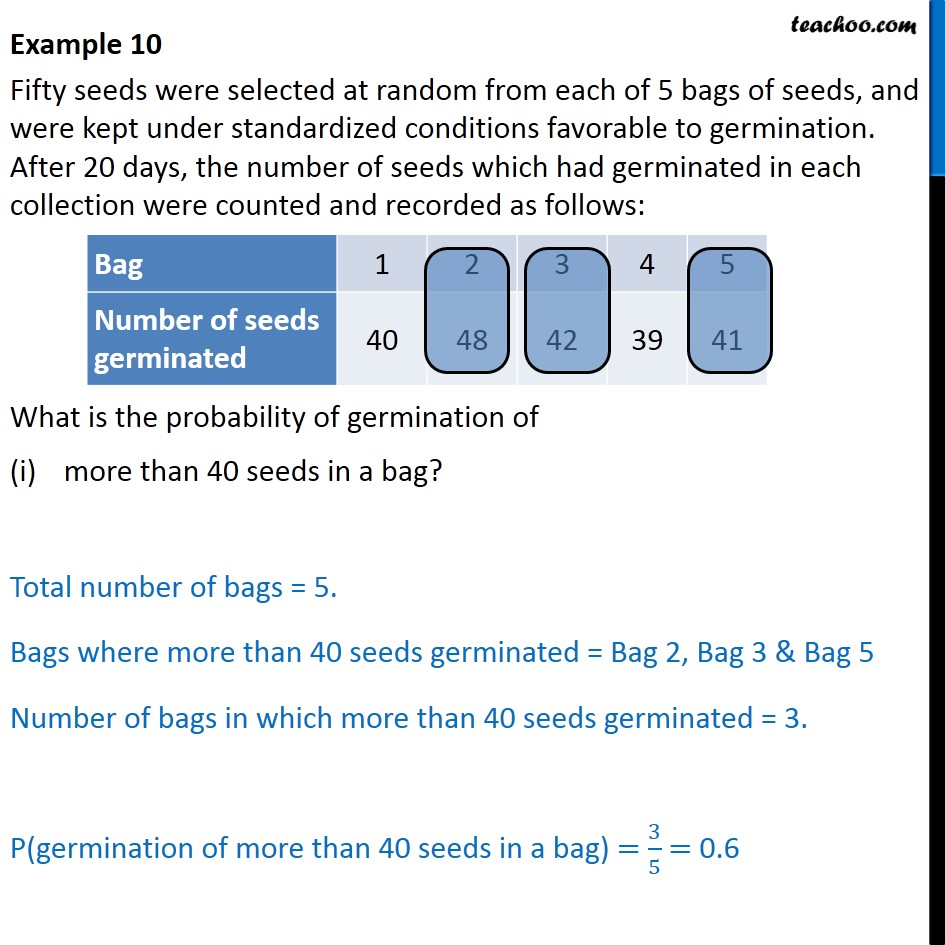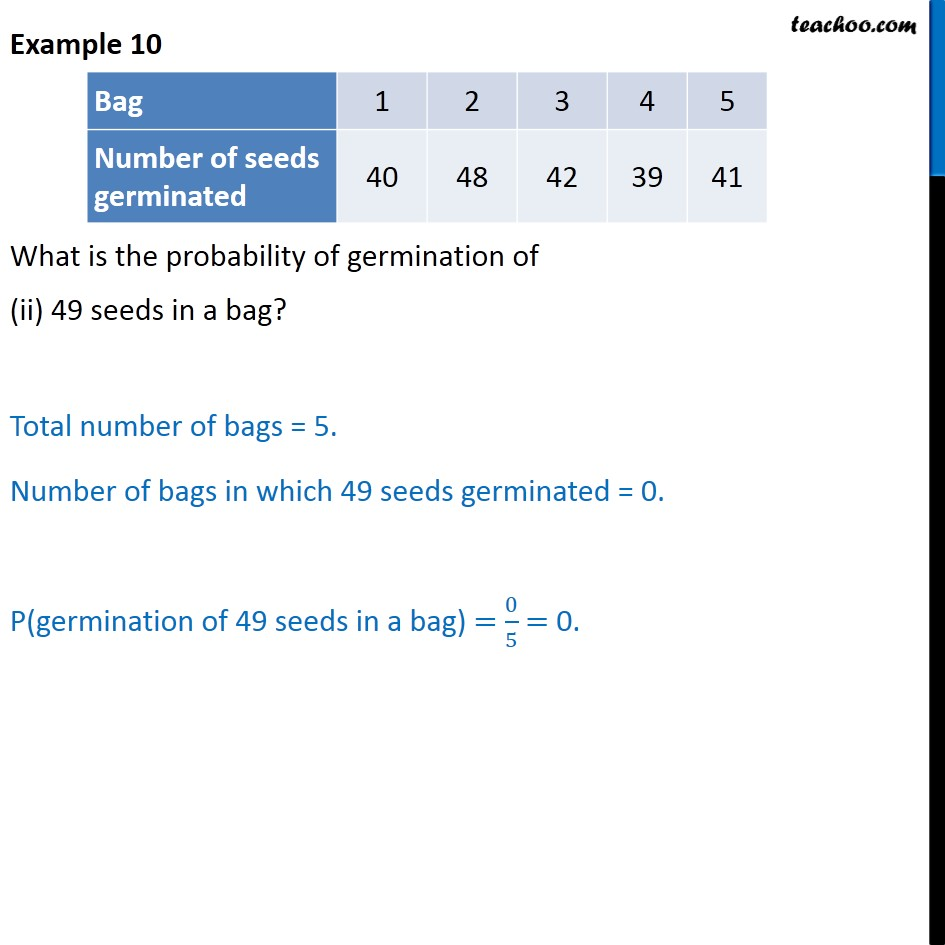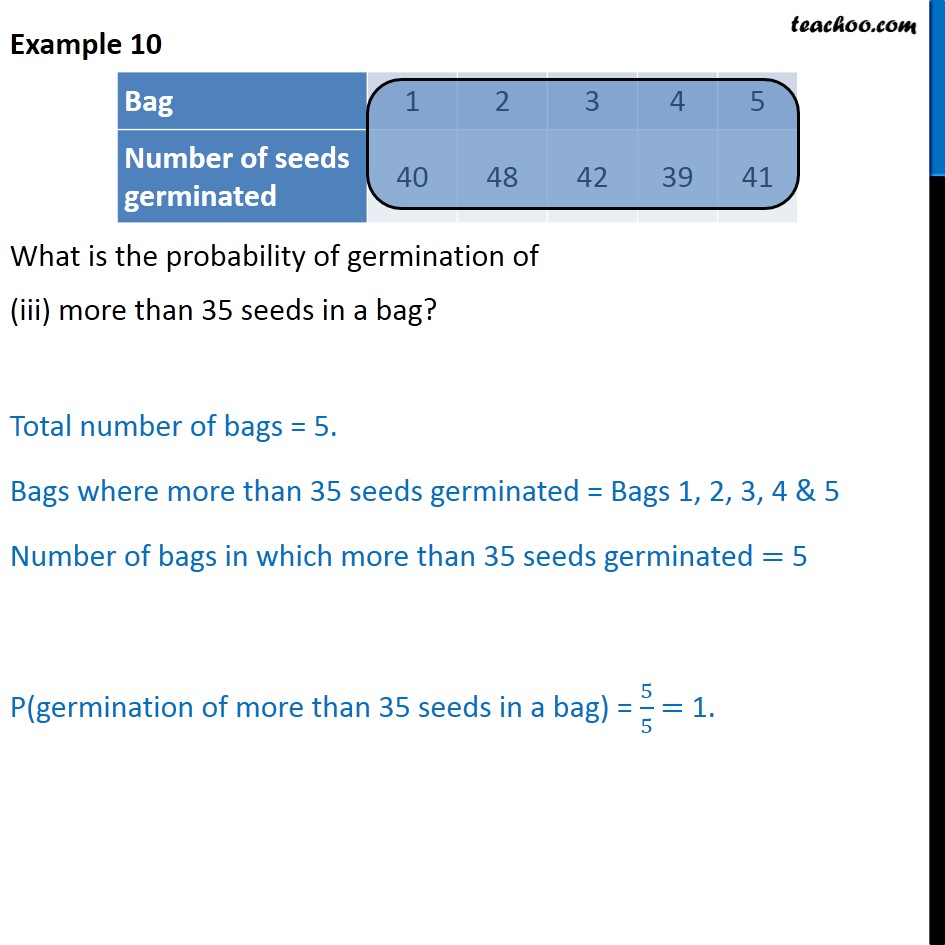Examples

Probability
Serial order wiseLearn in your speed, with individual attention - Teachoo Maths 1-on-1 Class

### Transcript

Example 10 Fifty seeds were selected at random from each of 5 bags of seeds, and were kept under standardized conditions favorable to germination. After 20 days, the number of seeds which had germinated in each collection were counted and recorded as follows: What is the probability of germination of more than 40 seeds in a bag? Total number of bags = 5. Bags where more than 40 seeds germinated = Bag 2, Bag 3 & Bag 5 Number of bags in which more than 40 seeds germinated = 3. P(germination of more than 40 seeds in a bag) = 3/5 = 0.6 Example 10 What is the probability of germination of (ii) 49 seeds in a bag? Total number of bags = 5. Number of bags in which 49 seeds germinated = 0. P(germination of 49 seeds in a bag) = 0/5 = 0. Example 10 What is the probability of germination of (iii) more than 35 seeds in a bag? Total number of bags = 5. Bags where more than 35 seeds germinated = Bags 1, 2, 3, 4 & 5 Number of bags in which more than 35 seeds germinated = 5 P(germination of more than 35 seeds in a bag) = 5/5 = 1.